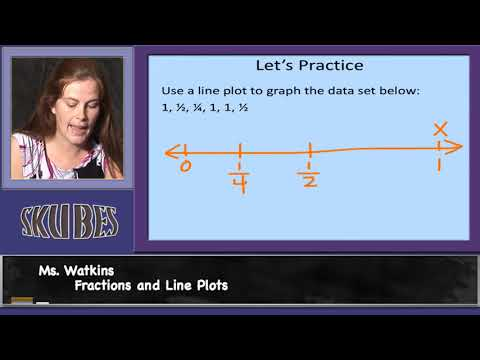# Fractions and Line Plots

##By Skubes ed

Use the four operations to solve word problems involving distances, intervals of time, liquid volumes, masses of objects, and money, including problems involving simple fractions or decimals, and problems that require expressing measurements given in a larger unit in terms of a smaller unit.# Motion - Introduction | Don't Memorise

##By Lumos Learning

In our earlier grades, we have studied motion and its types. Let’s have a quick review! Let’s start with motion. In simple words, if a body is moving, it’s described as motion!# 10th Grade Modeling with Systems of Equations

##By

10th grade math lesson on modeling with systems of equations. The lesson involved high school modeling standard A.CED.A.2 from the MA Curriculum Frameworks for Mathematics, which specifies creating equations in two or more variables.